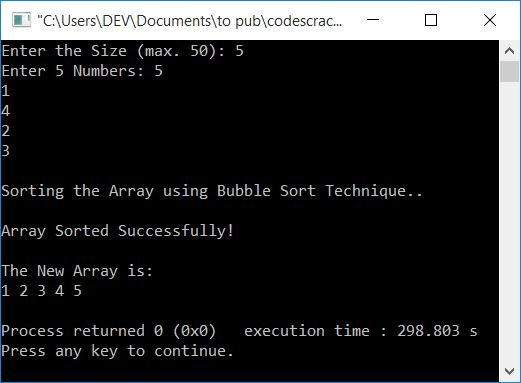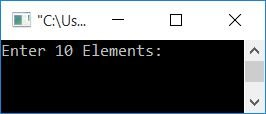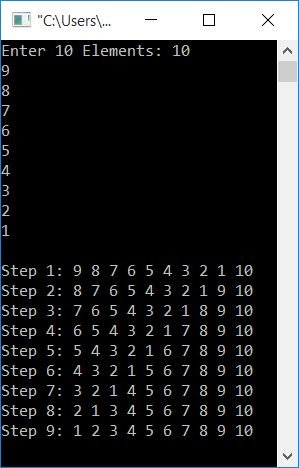# C++ Program for Bubble Sort

In this article, you will learn about and get code for implementing the bubble sort technique in a C++ program. That is, you'll get the code for how to sort an array in ascending order using the bubble sort technique. The bubble sort program is created with the following approaches:

But before starting the program, if you're not aware of it, you can follow bubble sort to understand its logic with an example.

## Bubble Sort in C++

To sort an array in ascending order using bubble sort in C++ programming, you have to ask the user to enter the array size and its elements. Now, use the bubble sort method to sort the array elements and show the sorted array on the screen, as shown in the next program.

```#include<iostream>
using namespace std;
int main()
{
int n, i, arr, j, temp;
cout<<"Enter the Size (max. 50): ";
cin>>n;
cout<<"Enter "<<n<<" Numbers: ";
for(i=0; i<n; i++)
cin>>arr[i];
cout<<"\nSorting the Array using Bubble Sort Technique..\n";
for(i=0; i<(n-1); i++)
{
for(j=0; j<(n-i-1); j++)
{
if(arr[j]>arr[j+1])
{
temp = arr[j];
arr[j] = arr[j+1];
arr[j+1] = temp;
}
}
}
cout<<"\nArray Sorted Successfully!\n";
cout<<"\nThe New Array is: \n";
for(i=0; i<n; i++)
cout<<arr[i]<<" ";
cout<<endl;
return 0;
}```

This program was built and runs under the Code::Blocks IDE. Here is the initial snapshot of the sample run:Now enter the size, say 5, and then its elements as 5, 1, 4, 2, 3. Press the ENTER key, and the list gets sorted in ascending order as shown in the final snapshot of the sample run given below:If the user enters the size of the array as 5, and its elements as 5, 1, 4, 2, 3, then the dry run of the above program goes like this:

• because the size is 5. Therefore, n=5
• And all the 5 elements gets initialized in the array arr[] in a way that arr = 5, arr = 1, arr = 4, arr = 2, and arr = 3.
• Using a for loop, initially i = 0 and checks whether it is less than n-1 or not.
• Because the condition (i<(n-1) or 0<(5-1) or 0<4) evaluates to be true, therefore program flow goes inside the loop
• Inside the loop, there is another for loop, and j is initially equal to 0, and the condition j<(n-i-1) or j<(5-0-1) or j<4 evaluates to be true. Therefore, program flow goes inside this loop.
• And checks the if block's condition, that is, arr[j]>arr[j+1] or arr>arr or 5>1 evaluates to true, program flow enters the if block and executes all three statements.
• After executing all three statements, the element at index j gets swapped with the element at index j+1.
• Now arr=1 and arr=5, and the rest of the three elements will be at the same index.
• Now program flow goes to the updating part of the inner for loop and increments the value of j. Now j=1
• Again checks the condition, that is j<(n-i-1) or 1<(5-0-1) or 1<4 evaluates to be true, therefore program flow again goes inside the loop and checks the condition of if block
• If the condition evaluates to be true, then process the three statements; otherwise, update the value of j and checks the condition.
• Continue in a similar manner with the updated value until the outer for loop condition evaluates to false.
• If the condition of the outer for loop evaluates to be false, then program flow exits the loop.
• Now that the array arr[] gets sorted, just print it.

### After each sort, print the array

Let's make another program that will dispel almost all of your concerns about bubble sort. This program prints the array after each sort. Take a deep look at all the arrays after each sort.

```#include<iostream>
using namespace std;
int main()
{
int i, arr, j, temp;
cout<<"Enter 10 Elements: ";
for(i=0; i<10; i++)
cin>>arr[i];
cout<<endl;
for(i=0; i<9; i++)
{
for(j=0; j<(10-i-1); j++)
{
if(arr[j]>arr[j+1])
{
temp = arr[j];
arr[j] = arr[j+1];
arr[j+1] = temp;
}
}
cout<<"Step "<<i+1<<": ";
for(j=0; j<10; j++)
cout<<arr[j]<<" ";
cout<<endl;
}
cout<<endl;
return 0;
}```

Here is the initial snapshot of the sample run:Now enter any 10 elements in random order to sort them in ascending order. Here I've entered 10 elements in descending order, say 10, 9, 8, 7, 6, 5, 4, 3, 2, 1. After supplying all 10 elements, press ENTER to get the output as given in the final snapshot here:Note: The last array, or the array at the last step (step no. 9), is a sorted array.

## Bubble Sort in C++ using a Function

This is the last program on bubble sort. This program is created using the function bubbleSort(). The function receives the array as its argument and sorts it in ascending order.

```#include<iostream>
using namespace std;
void bubbleSort(int []);
int main()
{
int i, arr;
cout<<"Enter 10 Elements: ";
for(i=0; i<10; i++)
cin>>arr[i];
bubbleSort(arr);
cout<<"\nThe New Sorted Array is: \n";
for(i=0; i<10; i++)
cout<<arr[i]<<" ";
cout<<endl;
return 0;
}
void bubbleSort(int arr[])
{
int i, j, temp;
for(i=0; i<9; i++)
{
for(j=0; j<(10-i-1); j++)
{
if(arr[j]>arr[j+1])
{
temp = arr[j];
arr[j] = arr[j+1];
arr[j+1] = temp;
}
}
}
}```

Here is its sample run:#### The same program in different languages

C++ Quiz

« Previous Program Next Program »